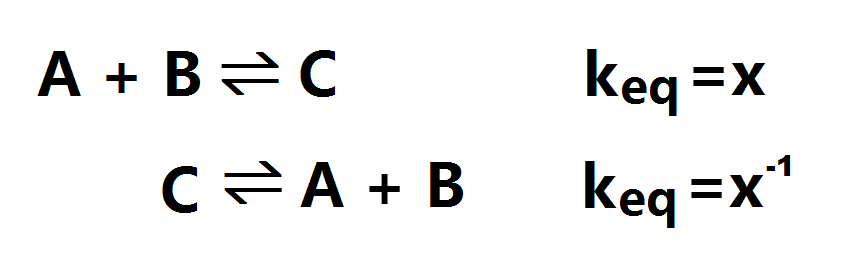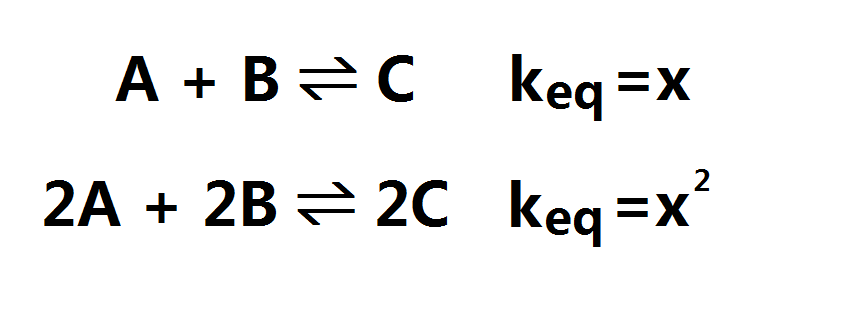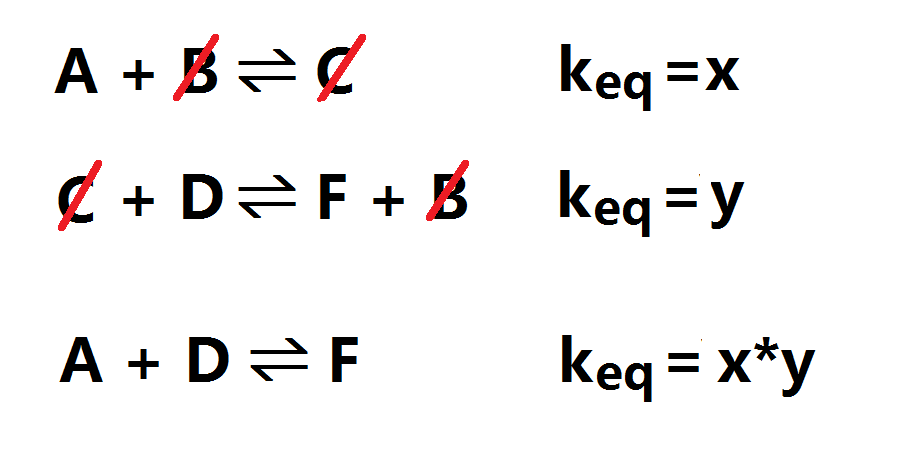Monday, November 21, 2011

Chemical Equilibrium #4: Manipulating the Equilibrium Constant (keq)

When calculating the keq of the reverse reaction:When multiplying the entire equation by a number:When adding several equations together: